Next: 7.2 Development Up: 7.1 Bake Steps Previous: 7.1.1 Prebake

## 7.1.2 Post-Exposure Bake

Depending on the resist system post-exposure bakes are performed to reduce standing wave effects or to thermally catalyze chemical reactions amplifying the latent bulk image. The former phenomenon is usually exploited in case of conventional DQN resists, whereas the latter forms the basis of chemically amplified resists. The following two models are suitable for one of the two resist systems.

Conventional resists. Many attempts have been made to reduce the vertically non-uniform exposure pattern that is caused by substrate reflections and leads to standing waves within the resist. One particularly useful method is a post-exposure or pre-development bake first proposed by Edward Walker . Both linewidth control and resolution are thereby significantly increased. The utilized physical phenomenon can be explained as the diffusion of the PAC within the film during the high temperature bake. Typical bake temperatures are about 100oC, and bake times tpeb range around 10 minutes in an oven.

For the modeling it usually suffices to assume a concentration independent diffusion coefficient Dpeb. This simple situation is called Fickean diffusion since the distribution of the PAC m(x;t) is governed by Fick's second law of diffusion that writes as [206, p. 71]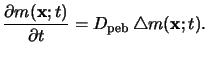(7.8)

Note that the above law is only valid for a constant diffusion coefficient Dpeb. The initial conditions are given by the calculated spatial distribution mexp(x) after exposure, i.e., (7.9) has to be solved subject to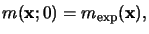(7.9)

and no out-diffusion of the PAC is assumed across the resist surface, i.e., homogeneous Neumann boundary conditions are imposed.

The solution of (7.9) is obtained with the help of the fundamental solutions mp(x;t) for an ideal unit impulse source'' located at any position xp inside the resist. These fundamental solutions mp(x;t) equal the Gaussian distribution function [206, p. 71](7.10)

whereby the standard deviation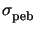is commonly called diffusion length.is related to the diffusion coefficient Dpeb and the bake time tpeb by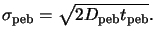(7.11)

Since the initial distribution mexp(x) can be written as superposition of weighted impulses like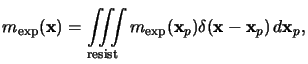(7.12)

and the integration and differentiation are interchangeable, the PAC distribution m(x;tpeb) after the post-exposure bake can be written as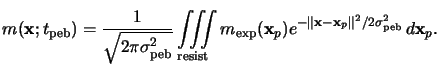(7.13)

This relation equals a convolution of the PAC concentration mexp(x) right after exposure with the Gaussian distribution function mp(x;t) of (7.11), i.e.,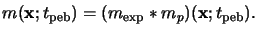(7.14)

For the numerical evaluation the integrations in (7.14) are approximated by summations over volume elements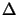x, whereby the grid spacing should be smaller than three times the diffusion length, e.g.,x3.

Only one parameter appears in the Fickean diffusion model (7.15), namely the diffusion lengthgiven in (7.12). This simple approach is physically limited since the PAC is the only considered component of the resist and therefore the reactions between the different resist compounds cannot be taken into account. However, it is the common model for the post-exposure bake step in case of conventional DQN-resists. For example, the widely used lithography simulators PROLITH , SAMPLE  and SOLID-C  rely on it.

Chemically amplified resists. In case of chemically amplified resists the reactions governing the bake effects have to be considered simultaneously with the diffusion of the involved species. An extension of the simple considerations presented in Section 5.1.3 was proposed by Richard Ferguson [87,88]. In this model two species are considered, namely reactive sites m(x;t) not consumed by the acid and the acid concentration h(x;t) produced during exposure. These species are assumed to react as follows: Firstly, the concentration  m(x;t) is driven by its availability and the acid concentration h(x;t) raised to a power n, and, secondly, the catalyzing acid h(x;t) decreases with time according to its concentration because of possible loss mechanism such as neutralization or time-sharing with the deprotected material. Note that the first reaction also occurs in the simple model (5.16), whereas the acid-loss mechanism corresponds to the extension. These two reactions are completed by a diffusion term for the acid h(x;t) yielding two coupled partial differential equations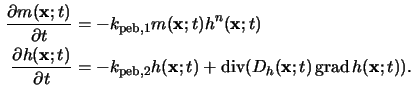The reaction order n and the first rate parameter kpeb, 1 characterize the chemical amplification (cf. (5.18)), whereas the second rate parameter kpeb, 2 describes potential loss mechanisms of the catalyzer. The diffusion coefficient Dh(x;t) of the acid depends on the already reacted, consumed sites x(x;t) = 1 - m(x;t). Various models have been proposed to describe this dependence, e.g.,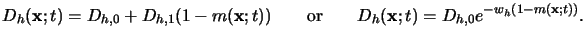(7.15)

The linear relationship reflects possible use of deprotected sites as stepping stones, and the exponential dependence accounts for free volume effects . All resist parameters typically exhibit an Arrhenius-type temperature behavior.

At the beginning of the bake no sites are activated and the acid concentration is obtained from the preceding exposure simulation. The initial conditions for (7.16) are thus given by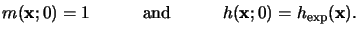(7.16)

The following boundary conditions are usually imposed: The resist/substrate interface can be assumed to be impermeable so that no diffusion occurs across it. At the lateral boundaries either periodic or homogeneous Neumann conditions are imposed, i.e., in the latter case a vanishing flux across the lateral boundaries is prescribed. The physically most important boundary segment is the resist surface, since acid diffusion into the atmosphere above the wafer occurs. The amount of this acid evaporation is a function of the acid size and the degree of its interaction with the resist polymer. A small acid molecule will have significant evaporation, for large molecules this effect will be negligible. In general, the evaporation is modeled by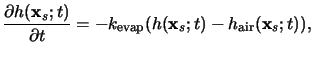(7.17)

whereby hair(xs;t) is the acid concentration in the atmosphere in the vicinity of the resist surface  xs. Usually, the post-exposure bake takes place in a reasonable open environment with enough air flow to eliminate any buildup of evaporated acid above the resist. Thus hair(xs;t) can be neglected. If kevap is very small, then virtually no evaporation takes place. On the other hand, if kevap is very large, the effect is to bring the acid surface concentration in the resist to zero.

This post-exposure bake model was successfully applied to study bake effects of various chemically amplified resist systems. Simulation parameters for the important IBM APEX-E resist are summarized in Table 7.1. A similar model was also used for a popular negative acid-hardening resist .

Table 7.1: Post-exposure bake parameters at 90oC for the IBM APEX-E chemically amplified resist.

 Parameter Equation Value Unit Ref. A (5.8) -0.001 1/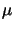m  B (5.8) 0.37 1/m  C (5.15) 0.0042 cm2/mJ  kpeb, 1 (7.16) 2.0 1/s  kpeb, 2 (7.16) 0.0033 1/s  n (7.16) 1.8 --  Dh, 0 (7.17) 0.0933 nm2/s  wh (7.17) 5.8 --Next: 7.2 Development Up: 7.1 Bake Steps Previous: 7.1.1 Prebake
Heinrich Kirchauer, Institute for Microelectronics, TU Vienna
1998-04-17